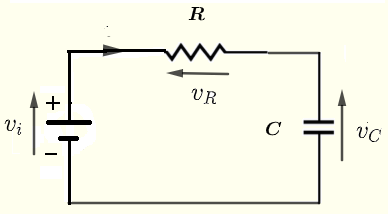# Step Response of a Series RC Circuit - Calculator

An online calculator to calculate and graph the current through and voltages across a resistor, a capacitor and an inductor in series when the input a step voltage of the form $V_0 u(t)$ where $u(t)$ is the unit step function.

## Formulae for Voltages and Current in a series RC Circuit to a Step Input Voltage

We first give the formulas used in the series RC calculator.The formulas developed in RC circuit response to a step voltage are presented here as they are used in the calculator.
When a voltage step function of the form $v_i(t) = V_0 u(t)$ is the input voltage in the given cicuit, we have :
The voltage $v_C(t)$ across the capacitor is given by
$v_C(t) = V_0 (1 - e^{-t/RC} ) u(t)$
The voltage $v_R(t)$ across the resistor is given by
$v_R (t) = V_0 e^{-t/RC} u(t)$
The current $i(t)$ is given by
$i(t) = \dfrac{v_R}{R} = \dfrac{V_0}{R} e^{-t/RC} u(t)$
$\tau = R C$ is called the time constant of the circuit.

## Use of the calculator

Enter the source voltage $V_0$, the resistance $R$, the capacitance $C$ as positive real numbers with the given units then press "Calculate".
The time constant $\tau$, the expressions of the voltages $v_C(t)$ and $v_R(t)$ and their graphs and the expression of the current $i(t)$ are displayed.

 Source Voltage $V_0$ = 1 V Resistance R = 100 mΩ Ω KΩ MΩ Capacitance C = 100 pF nF μF mF F Number of Significant Digits = 3

## Results

### More References and links

series RLC circuit response to a step voltage
Engineering Mathematics with Examples and Solutions